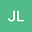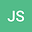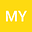Global large solutions for the Navier-Stokes equations with the Coriolis forc
•••• Jinlu Li,
• Jinyi Sun,
• Minghua Yang
Jinlu Li
School of Mathematics and Computer Sciences
Author ProfileJinyi Sun
Northwest Normal University
Author ProfileMinghua Yang
Jiangxi University of Finance and Economics
Author Profile## Abstract

In this paper, we construct a class of global large solution to the three-dimensional Navier-Stokes equations with the Coriolis force in critical Fourier-Besov space $\dot{FB}^{2-\frac{3}{p}}_{p,r}(\mathbb{R}^3)$. In fact, our choice of special initial data $u_0$ can be arbitrarily large in $\dot{FB}^{s}_{p,r}(\mathbb{R}^3)$ for any $s\in\R$ and $1\leq p,r\leq \infty$.